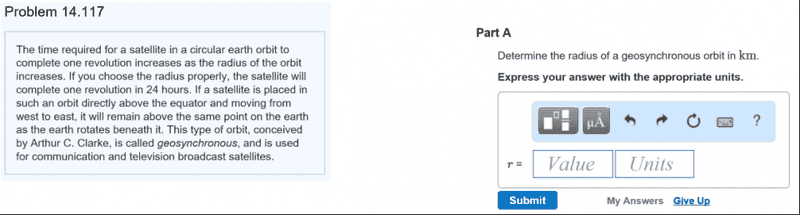# Earth orbit -- geosynchronous orbit calculation

nysnacc

## Homework Statement## Homework Equations

ε= r_max - r_min / r_max + r_min
= r*v2 / gRE

## The Attempt at a Solution

I first find that 1 revolution is 2π rad , the angular velocity ω = 2π rad / 24h = π/43200 rad /s
so v = rω where r is the unknown
ε = 0 (circular orbit)

r*(rω)2 / g RE = 0

Correct route?

## Answers and Replies

Homework Helper
Gold Member
2021 Award
Your approach is incorrect. You need to use Newton's 2nd law to find a relation between speed and radius in a circular orbit.

nysnacc
st
Your approach is incorrect. You need to use Newton's 2nd law to find a relation between speed and radius in a circular orbit.

Homework Helper
Gold Member
2021 Award
No need for integration. Remember that the acceleration here is centripetal. What is an expression for F?

nysnacc
F=ma then a is gravity?

Homework Helper
Gold Member
2021 Award
No, a is the centripetal acceleration. What is an expression for it? F is the force of gravitational attraction between two masses according to Newton's law of gravitational attraction.

nysnacc
GmM/ r2

Homework Helper
Gold Member
2021 Award
That's F. What about a on the other side of the equation?

nysnacc
m (r'' + rω) cuz it is the angular movement ?